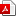# SIMULTANEOUS ESTIMATION OF PRODUCTION FUNCTION AND BENEFICIARIES OF GROWTH FOR A DEVELOPING ECONOMY

### Abstract

In this paper we have identified the sources of growth and its beneficiaries in Pakistan’s large scale manufacturing sector using a simultaneous equation model. The use of such a model was necessitated since the Sims Test revealed endogeneity in the variables. We find the single equation estimates biased when compared with the ones obtained from the use of the simultaneous model. Using the latter estimates we find the contribution of capital much less, and the contribution of labor almost double as compared with the single equation estimates. The contribution of technological change declines just a little. The single equation estimates revealed constant returns to scale, while the simultaneous model indicated the presence of increasing returns. We find that every one per cent increase in productivity leads to a 0.6 per cent increase in wages and a 0.6 per cent decline in prices.VIEW PDF